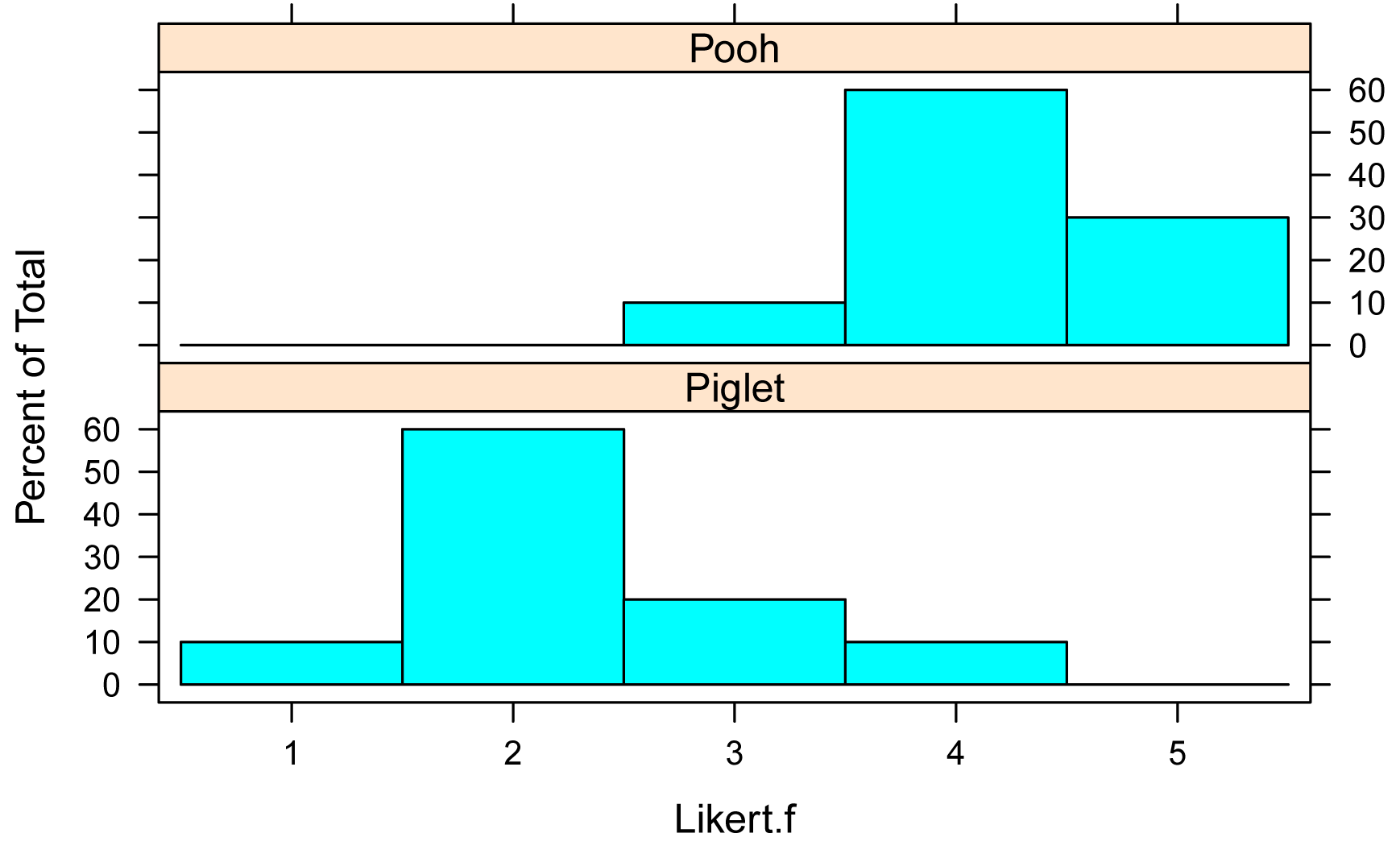## Summary and Analysis of Extension Program Evaluation in R

Salvatore S. Mangiafico

# Two-sample Ordinal Test with CLM

##### Appropriate data

•  Two-sample data.  That is, one-way data with two groups only

•  Dependent variable is ordered factor

•  Independent variable is a factor with two levels.  That is, two groups

•  Observations between groups are independent.  That is, not paired or repeated measures data

##### Interpretation

A significant result can be interpreted as, “There was a significant difference between groups.”  Or, “There was a significant effect of Independent Variable.”

### Packages used in this chapter

The packages used in this chapter include:

•  psych

•  FSA

•  lattice

•  ordinal

•  car

•  RVAideMemoire

The following commands will install these packages if they are not already installed:

if(!require(psych)){install.packages("psych")}
if(!require(FSA)){install.packages("FSA")}
if(!require(lattice)){install.packages("lattice")}
if(!require(ordinal)){install.packages("ordinal")}
if(!require(car)){install.packages("car")}
if(!require(RVAideMemoire)){install.packages("RVAideMemoire")}

### Two-sample ordinal model example

Input =("
Speaker  Likert
Pooh      3
Pooh      5
Pooh      4
Pooh      4
Pooh      4
Pooh      4
Pooh      4
Pooh      4
Pooh      5
Pooh      5
Piglet    2
Piglet    4
Piglet    2
Piglet    2
Piglet    1
Piglet    2
Piglet    3
Piglet    2
Piglet    2
Piglet    3
")

### Create a new variable which is the Likert scores as an ordered factor

Data\$Likert.f = factor(Data\$Likert,
ordered = TRUE)

###  Check the data frame

library(psych)

str(Data)

summary(Data)

### Remove unnecessary objects

rm(Input)

#### Summarize data treating Likert scores as factors

xtabs( ~ Speaker + Likert.f,
data = Data)

Likert.f
Speaker  1 2 3 4 5
Piglet 1 6 2 1 0
Pooh   0 0 1 6 3

XT = xtabs( ~ Speaker + Likert.f,

data = Data)

prop.table(XT,
margin = 1)

Likert.f
Speaker    1   2   3   4   5
Piglet 0.1 0.6 0.2 0.1 0.0
Pooh   0.0 0.0 0.1 0.6 0.3

#### Bar plots of data by group

library(lattice)

histogram(~ Likert.f | Speaker,
data=Data,
layout=c(1,2)      #  columns and rows of individual plots
)#### Summarize data treating Likert scores as numeric

library(FSA)

Summarize(Likert ~ Speaker,
data=Data,
digits=3)

Speaker  n mean    sd min Q1 median   Q3 max percZero
1  Piglet 10  2.3 0.823   1  2      2 2.75   4        0
2    Pooh 10  4.2 0.632   3  4      4 4.75   5        0

#### Two-sample ordinal model example

The model is specified using formula notation.  Here, Likert.f is the dependent variable and Speaker is the independent variable.  The data= option indicates the data frame that contains the variables.  For the meaning of other options, see ?clm.

The p-value for the effect of Speaker is determined using the Anova function in the packages RVAideMemoire and car.

##### Define model

library(ordinal)

model = clm(Likert.f ~ Speaker,
data = Data)

##### Analysis of deviance

library(car)

library(RVAideMemoire)

Anova(model,
type = "II")

Analysis of Deviance Table (Type II tests)

LR Chisq Df Pr(>Chisq)
Speaker   17.505  1  2.866e-05 ***

##### Check model assumptions

nominal_test(model)

Tests of nominal effects

formula: Likert.f ~ Speaker
Df  logLik    AIC LRT Pr(>Chi)
<none>     -20.199 50.397
Speaker

### No p-value produced for this example.

scale_test(model)

Tests of scale effects

formula: Likert.f ~ Speaker
Df  logLik    AIC    LRT Pr(>Chi)
<none>     -20.199 50.397
Speaker  1 -20.148 52.295 0.1019   0.7496

### No violation of model assumptions